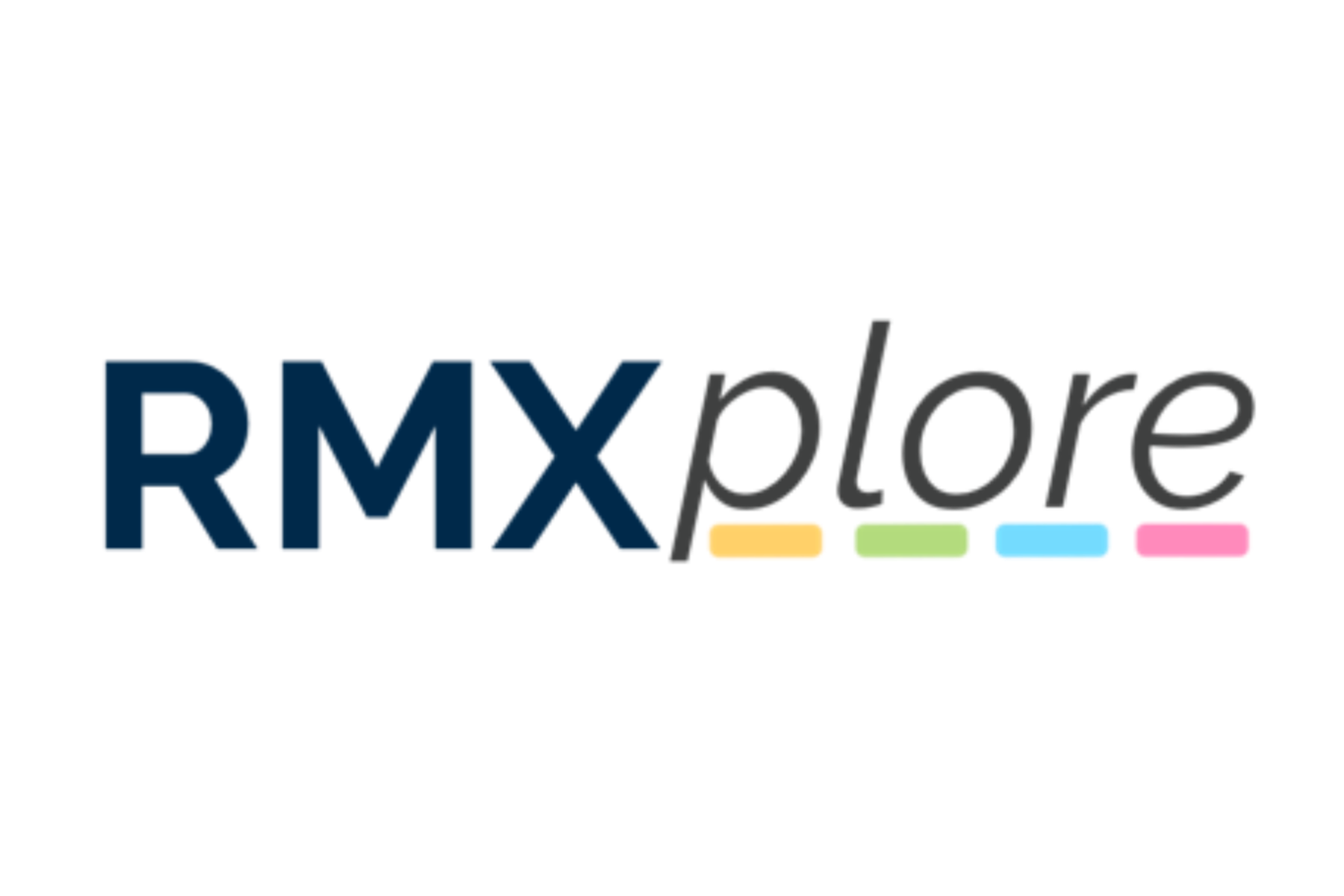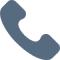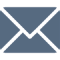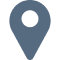# Algebra I

Please note that RMXplore's Math Bytes Courses are not
currently open for registration
.

### Algebra I

Coming Soon!
Course Pricing:
• Online : \$260
• Houston Center : \$320
• Cupertino Center : \$400
Course Logistics:
Duration : to be updated
Number of Classes: 5 weeks course
Occurs : to be updated

### Math Byte Course Topics:

Course Topics are organized as six modules with five granular topics covered under each module. Click through below to see all modules/topics for this course.

#### 01 - Numbers and Expressions• Writing Down Equations
• Simple 1-Variable Equations
• Graphing Linear Equations
• Application to Data

#### 02 - Linear Equations• Writing Down Equations
• Simple 1-Variable Equations
• Graphing Linear Equations
• Application to Data

#### 03 - Systems and Inequalities• Basic Inequality Rules
• Solving Linear Inequalities
• Solving Systems with Substitution
• Solving Systems with Elimination
• Graphing Systems and Inequalities

#### 04 - Exponents, Radicals, and Polynomials• Simplifying Expressions with Exponents
• Exponential Growth
• Radicals and Rationalizing Polynomials Operations
• Polynomial Equations• Completing the Squares
• Imaginary Numbers
• Discriminant and Applications of Quadratics

#### 06 - Statistics and Probability• Organizing and Representing Data
• Interpreting Plots of Data
• Measures of Central Tendencies and Dispersion
• Basics of Probability
• Rules of Probability